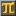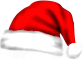#SCIENTIFIC CALCULATORThis calculation: Edit - Share - Print -
Save

• Press Ctrl-d to save this calculation as a bookmark.

- Reset

 sin(0.02) - 0.02 =   -0.00000133330666692 …  How many terms of Maclaurin series do I need to calculate sin(x)-x at x=0.02 with the same result? First term: sin(0) - 0 =   0.0  Plus second term: ans + (cos(0) - 1) * 0.02 =   0.0  Plus third term: ans + ( - sin(0)) / 2 * 0.02**2 =   0.0  Plus fourth term: ans - cos(0) / fact(3) * 0.02**3 =   -0.00000133333333333 …  Odd terms have sin(0) therefore they can be skipped. Plus sixth term: ans + cos(0) / fact(5) * 0.02**5 =   -0.00000133330666667 …  Plus eighth term: ans - cos(0) / fact(7) * 0.02**7 =   -0.00000133330666692 …  Eight terms! So with eight terms, what is the error?  -  =   1.41092961361E-21 …  Of course much of this error is the 10th term: cos(0) / fact(9) * 0.02**9 =   1.41093474427E-21 … 
CalculatorPi.comⒸ Troymius LLC